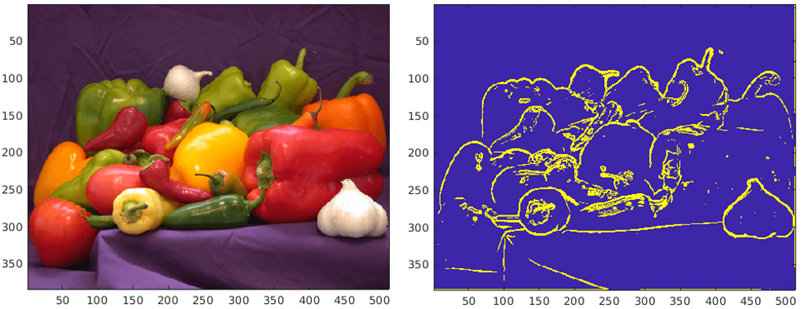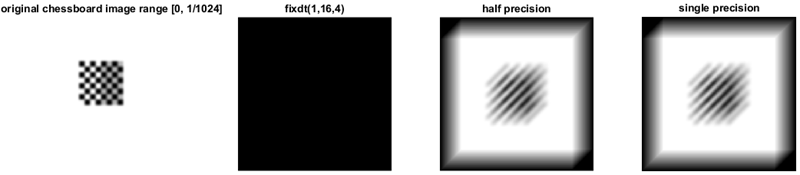## What is Half Precision?

The IEEE® 754 half-precision floating-point format is a 16-bit word divided into a 1-bit sign indicator s, a 5-bit biased exponent e, and a 10-bit fraction f.Because numbers of type `half` are stored using 16 bits, they require less memory than numbers of type `single`, which uses 32 bits, or `double`, which uses 64 bits. However, because they are stored with fewer bits, numbers of type `half` are represented to less precision than numbers of type `single` or `double`.

The range, bias, and precision for supported floating-point data types are given in the table below.

Data Type

Low Limit

High Limit

Exponent Bias

Precision

Half

2−14 ≈ 6.1·10−5

(2−2-10) ·215≈ 6.5·104

15

2−10 ≈ 10−3

Single

2−126 ≈ 10−38

2128 ≈ 3 · 1038

127

2−23 ≈ 10−7

Double

2−1022 ≈ 2 · 10−308

21024 ≈ 2 · 10308

1023

2−52 ≈ 10−16

For a video introduction to the half-precision data type, see What Is Half Precision? and Half-Precision Math in Modeling and Code Generation.

### Half Precision Applications

When an algorithm contains large or unknown dynamic ranges (for example integrators in feedback loops) or when the algorithm uses operations that are difficult to design in fixed-point (for example `atan2`), it can be advantageous to use floating-point representations. The half-precision data type occupies only 16 bits of memory, but its floating-point representation enables it to handle wider dynamic ranges than integer or fixed-point data types of the same size. This makes half precision particularly suitable for some image processing and graphics applications. When half-precision is used with deep neural networks, the time needed for training and inference can be reduced. By using half precision as a storage time for lookup tables, the memory footprint of the lookup table can be reduced.

#### MATLAB Examples

• Fog Rectification (GPU Coder) — The fog rectification image processing algorithm uses convolution, image color space conversion, and histogram-based contrast stretching to enhance the input image. This example shows how to generate and execute CUDA® MEX with half-precision data types for these image processing operations.• Edge Detection with Sobel Method in Half-Precision (GPU Coder) — The sobel edge detection algorithm takes an input image and returns an output image that emphasizes high spatial frequency regions that correspond to edges in the input image. This example shows how to generate and execute CUDA MEX with the half-precision data type used for the input image and Sobel operator kernel values.• Generate Code for Sobel Edge Detection That Uses Half-Precision Data Type (MATLAB Coder) — This example shows how to generate a standalone C++ library from a MATLAB® function that performs Sobel edge detection of images by using half-precision floating point numbers.

• Half-Precision Field-Oriented Control Algorithm — This example implements a Field-Oriented Control (FOC) algorithm using both single precision and half precision.

• Image Quantization with Half-Precision Data Types — This example shows the effects of quantization on images. While the fixed-point data type does not always produce an acceptable results, the half-precision data type, which uses the same number of bits as the fixed-point data type, produces a result comparable to the single-precision result.• Digit Classification with Half-Precision Data Types — This example compares the results of a trained neural network classification model in double precision and half precision.

• Convert Single Precision Lookup Table to Half Precision — This example demonstrates how to convert a single-precision lookup table to use half precision. Half precision is the storage type; the lookup table computations are performed using single precision. After converting to half precision, the memory size of the Lookup Table blocks are reduced by half while maintaining the desired system performance.

### Benefits of Using Half Precision in Embedded Applications

The half precision data type uses less memory than other floating-point types like single and double. Though it occupies only 16 bits of memory, its floating-point representations enables it to handle wider dynamic ranges than integer or fixed-point data types of the same size.

#### FPGA

The half precision data type uses significantly less area and has low latency compared to the single precision data type when used on hardware. Half precision is particularly advantageous for low dynamic range applications.

The following plot shows the advantage of using half precision for an implementation of a field-oriented control algorithm in Xilinx® Virtex® 7 hardware.#### GPU

In GPUs that support the half-precision data type, arithmetic operations are faster as compared to single or double precision.

In applications like deep learning, which require a large number of computations, using half precision can provide significant performance benefits without significant loss of precision. With GPU Coder™, you can generate optimized code for prediction of a variety of trained deep learning networks from the Deep Learning Toolbox™. You can configure the code generator to take advantage of the NVIDIA® TensorRT high performance inference library for NVIDIA GPUs. TensorRT provides improved latency, throughput, and memory efficiency by combining network layers and optimizing kernel selection. You can also configure the code generator to take advantage TensorRT's precision modes (FP32, FP16, or INT8) to further improve performance and reduce memory requirements.

#### CPU

In CPUs that support the half-precision data type, arithmetic operations are faster as compared to single or double precision. For ARM® targets that natively support half-precision data types, you can generate native half C code from MATLAB or Simulink®. See Code Generation with Half Precision.

### Half Precision in MATLAB

Many functions in MATLAB support the half-precision data type. For a full list of supported functions, see `half`.

Signals and block outputs in Simulink can specify a half-precision data type. The half-precision data type is supported for simulation and code generation for parameters and a subset of blocks. To view the blocks that support half precision, at the command line, type:

`showblockdatatypetable`

Blocks that support half precision display an `X` in the column labeled Half. For detailed information about half precision support in Simulink, see The Half-Precision Data Type in Simulink.

### Code Generation with Half Precision

The half precision data type is supported for C/C++ code generation, CUDA code generation using GPU Coder, and HDL code generation using HDL Coder™. For GPU targets, the half-precision data type uses the native half data type available in NVIDIA GPU for maximum performance.

For detailed code generation support for half precision in MATLAB and Simulink, see Half Precision Code Generation Support and The Half-Precision Data Type in Simulink.

For embedded hardware targets that natively support special types for half precision, such as `_Float16` and `_fp16` data types for ARM compilers, you can generate native half precision C code using Embedded Coder® or MATLAB Coder™. For more information, see Generate Native Half-Precision C Code from Simulink Models and Generate Native Half-Precision C Code Using MATLAB Coder.